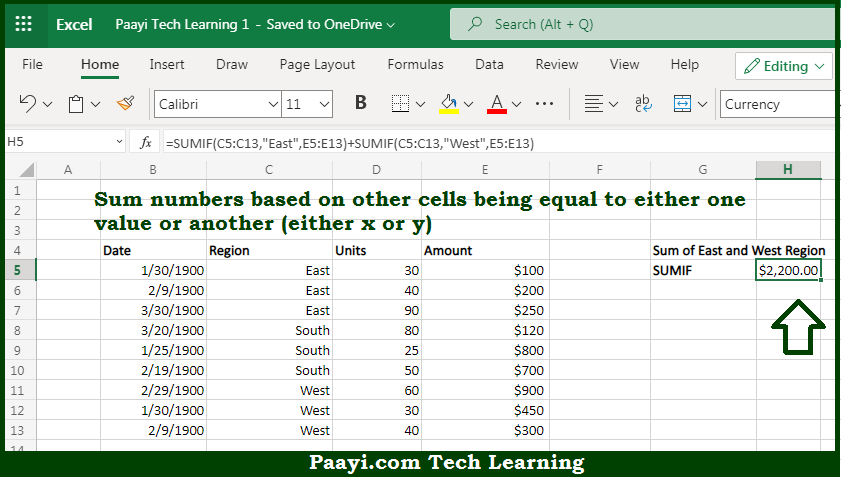# Learn How to SUM If Equal to Either x or y in Microsoft Excel

Written by | 0 Comments | 512 Views

In this article, you will learn how to SUM various things in Microsoft Excel using a single/combination(s) of functions. You will also know how to SUM If Equal to Either x or y and see the generic formula.

SUM If Equal to Either x or y in Microsoft Excel

The main purpose of this formula is to sum numbers based on other cells being equal to either one value or another. Here we will learn how to sum numbers either equal to x or y in the workbook in Microsoft Excel. That implies, with the help of formula based on the SUMIF function you can able to sum numbers based on other cells being equal to either one value or another. So, with the help of this formula, you can able to sum numbers either equal to x or y in the workbook in Microsoft Excel.

General Formula to SUM If Equal to Either x or y

=SUMIF(region,"West",amount)+SUMIF(region,"North",amount)

The Explanation for the SUM If Equal to Either x or ySo we know that with the help of the given formula above you can able to sum if cells end with specific text. Here we will learn how to sum if the date is ending with some specific text in the workbook in Microsoft Excel. As we know that the SUMIF function supports  logical operators efficiently, which are -  "=",">",">=", etc. So you can able to use these operators as per your criteria. So, with the help of this formula, you can able to sum if the date is ending with some specific text in the workbook in Microsoft Excel.